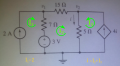# nodal analysis

#### e-learner

Joined Apr 25, 2015
30

#### shteii01

Joined Feb 19, 2010
4,644
One of the initial assumptions is that all mesh currents are in the same direction.
You, the user, choose the direction. Either clockwise or counter clock wise.

Your Mesh 1 current is 2 A, you chose clockwise.
Your Mesh 2 current is i1, you chose clockwise.
Your Mesh 3 current is 4(i1), you chose counter clock wise, mistake.

#### e-learner

Joined Apr 25, 2015
30
evenif I assume the current in clockwise direction , it will turn out to be positive. Is it not?
-3+7(i1-2)+15(i1)+5(i1-(-4i1))0
=>-3+7(i1-2)+15(i1)+5(i1+4i1)=0

#### The Electrician

Joined Oct 9, 2007
2,916
how to calculate v1 and v2 using mesh analysis . I was able to solve using nodal analysis but when i tried solving using mesh analysis I could not. I made following equation:
-3+7(i1-2)+15(i1)+5(i1+4i1)=0 (considering i1 as the mesh current)
and then v2=i1*5 and v1-v2=i1*15.

Is there some problem with the equations?View attachment 92880
I see 3 meshes but only 1 mesh equation. I would label the mesh currents I1, I2 and I3 starting with the leftmost mesh current as I1. Can you write 3 mesh equations? Also, what did you get for V1 and V2 using nodal analysis?

#### RBR1317

Joined Nov 13, 2010
706
Indeed, there are 3 meshes but only 1 mesh equation. Two of the meshes must provide constraint equations because one does not sum voltage drops across a current source. The sum of the mesh currents through a current source must equal that source of current. Also, though it is customary to have all mesh currents turn in the same direction, it is not required. Note that the variable 'i' will not appear in the mesh equation.Last edited:
•e-learner

#### The Electrician

Joined Oct 9, 2007
2,916
Indeed, there are 3 meshes but only 1 mesh equation. Two of the meshes must provide constraint equations because one does not sum voltage drops across a current source. The sum of the mesh currents through a current source must equal that source of current. Also, though it is customary to have all mesh currents turn in the same direction, it is not required. Note that the variable 'i' will not appear in the mesh equation.
View attachment 92901
The philosophy of the homework help forum is to guide the TS to the answer without giving too much of the answer itself. My intent here was to get the TS to recognize that 3 equations are needed. Part of what he may not have encountered before is a mesh including a current source, and if in the process of trying to write mesh equations, he realizes that mesh 1 and 3 need different treatment than summing voltages, we will have an opportunity to guide him. I think you may be going too far, too soon. You gave him the equation for mesh 1, without giving him a chance to derive it himself.

In another thread, you gave the TS a solution while he (she?) was still in the process of working it out:

It might be useful to a student of network theory to realize that the mesh method consists in finding equations that constrain the current in each mesh, whether by constraining the sum of voltages around the loop to be zero, or something else. Realizing when that "something else" is needed is part of the process.

#### e-learner

Joined Apr 25, 2015
30
hey, I posted wrong in my question. I actually calculated v2=(i1+4i1)*5.
I had tried both mesh analysis and nodal analysis( I have attached the images). But I am getting wrong answer using mesh analysis. PLz help.

#### e-learner

Joined Apr 25, 2015
30
I found my mistake . I was calculating the dependent current source value in a wrong way. Ignore the above attached image of mesh analysis.Thank you so much.#### e-learner

Joined Apr 25, 2015
30
Indeed, there are 3 meshes but only 1 mesh equation. Two of the meshes must provide constraint equations because one does not sum voltage drops across a current source. The sum of the mesh currents through a current source must equal that source of current. Also, though it is customary to have all mesh currents turn in the same direction, it is not required. Note that the variable 'i' will not appear in the mesh equation.
View attachment 92901
Indeed, there are 3 meshes but only 1 mesh equation. Two of the meshes must provide constraint equations because one does not sum voltage drops across a current source. The sum of the mesh currents through a current source must equal that source of current. Also, though it is customary to have all mesh currents turn in the same direction, it is not required. Note that the variable 'i' will not appear in the mesh equation.
View attachment 92901
Thank you so much.Although you give a hint but it took me a couple of hours to find my mistake. This problem was troubling me for days. At last, I learned something.